JMSLTM Numerical Library 7.2.0
com.imsl.datamining.neural

## Class TimeSeriesClassFilter

• All Implemented Interfaces:
Serializable

```public class TimeSeriesClassFilter
extends Object
implements Serializable```
Converts time series data contained within nominal categories to a lagged format for processing by a neural network. Lagging is done within the nominal categories associated with the time series.

Class `TimeSeriesClassFilter` can be used with a data array, `x[]` to compute a new data array, z[][], containing lagged columns of `x[]`.

When using the method `computeLags`, the output array, z[][] of lagged columns, can be symbolically represented as: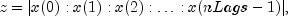where x(i) is a lagged column of the incoming data array `x`, and nLags is the number of computed lags. The lag associated with x(i) is equal to the value in `lag[i]`, and lagging is done within the nominal categories given in `iClass[]`. This requires the time series data in `x[]` be sorted in time order within each category `iClass`.

Consider an example in which the number of observations in `x[]` is 10. There are two lags requested in `lag[]`. If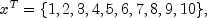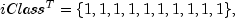and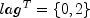then, all the time series data fall into a single category, i.e. `nClasses` = 1, and z would contain 2 columns and 10 rows. The first column reproduces the values in `x[]` because `lags`=0, and the second column is the 2nd lag because `lags`=2.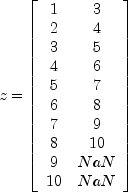On the other hand, if the data were organized into two classes with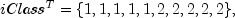then `nClasses` is 2, and z is still a 2 by 10 matrix, but with the following values: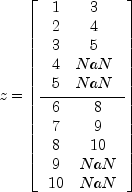The first 5 rows of z are the lagged columns for the first category, and the last five are the lagged columns for the second category.

Example, Serialized Form
• ### Constructor Summary

Constructors
Constructor and Description
`TimeSeriesClassFilter(int nClasses)`
Constructor for `TimeSeriesClassFilter`.
• ### Method Summary

Methods
Modifier and Type Method and Description
`double[][]` ```computeLags(int[] lags, int[] iClass, double[] x)```
Computes lags of an array sorted first by class designations and then descending chronological order.
• ### Methods inherited from class java.lang.Object

`clone, equals, finalize, getClass, hashCode, notify, notifyAll, toString, wait, wait, wait`
• ### Constructor Detail

• #### TimeSeriesClassFilter

`public TimeSeriesClassFilter(int nClasses)`
Constructor for `TimeSeriesClassFilter`.
Parameters:
`nClasses` - An `int` specifying the number of nominal categories associated with the time series.
• ### Method Detail

• #### computeLags

```public double[][] computeLags(int[] lags,
int[] iClass,
double[] x)```
Computes lags of an array sorted first by class designations and then descending chronological order.
Parameters:
`lags` - An `int` array containing the requested lags. Every lag must be non-negative.
`iClass` - An `int` array containing class number associated with each element of `x`, sorted in ascending order. The i-th element is equal to the class associated with the i-th element of `x`. `iClass` and `x` must be the same length.
`x` - A `double` array containing the time series data to be lagged. This array is assumed to be sorted first by class designations and then descending chronological order, i.e., most recent observations appear first within a class.
Returns:
A `double` matrix containing the lagged data. The i-th column of this array is the lagged values of `x` for a lag equal to `lags[i]`. The number of rows is equal to the length of `x`.
JMSLTM Numerical Library 7.2.0